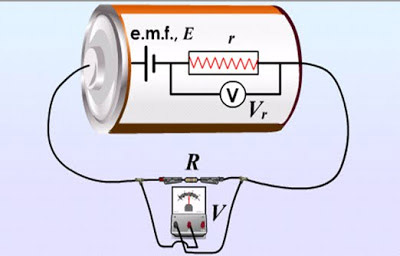A# electromotive forceThe electromotive force of this battery is its terminal voltage less the voltage across the internal resistance (Vr= Ir). There may also be voltage drops across components of the external circuit (such a the voltage V across the resistor R in the case shown).

Electromotive force (emf) is the amount of energy that a unit charge can gain in passing through a cell or a battery. It is not a force at all but instead is the energy that can be transferred to a charge because of a difference in electrical potential between the two electrodes of a cell. Electromotive force is analogous to the potential energy a mass acquires by virtue of a difference in height between two points in a gravitational field. Electromotive force is measured in volts (= joules per coulomb).

Emf is not the same thing as voltage, even though both are measured in volts. Voltage is the potential difference between any two given points in a circuit. So, for example, we might talk about the "voltage across a resistor" when referring to the drop in electrical potential energy across that component. The voltage between any two points in a circuit will always be less than the emf. In fact, even the terminal voltage, the difference in potential energy between the two electrodes of a cell, will be less than the emf when a current is flowing because some energy must be used in overcoming the internal resistance of the cell. Applying Ohm's law internally to the cell, we can say that:

V = E – Ir

where V is terminal voltage, E is the emf, I is the current, and r is the internal resistance.

## emf and conventional current flow

Although, in circuits, electrical current is actually a flow of electrons, which are negatively charged, for historical reasons 'conventional current' is taken to be a flow of positive charge from the positive to the negative terminal. As a result of this tradition, emf is defined to be the energy expended, or work done, in moving a unit positive charge through a closed circuit, including the cell itself. Conventional current flows from higher to lower potential outside the cell and from lower to higher potential inside the cell. No work needs to be done when current flows from higher to lower potential, but inside the cell, work needs to be done in order to move the positive charge from a lower to higher potential.

## emf due to induction

So far, we have referred to electromotive force in the context of two-terminal devices such as an electrochemical cell or battery but emfs can also be generated in other ways, for example by electromagnetic induction. A varying magnetic field will induce an emf in a circuit. The size of this emf is determined by the rate of change of the magnetic flux through the circuit. The production of an emf in an electrical conductor by a varying magnetic field is the basic operating principle of many types of electric motors and generators, as well as transformers and inductors.